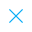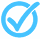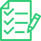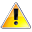Call Support +91-85588-96644

Keep me logged in
You can't leave Captcha Code empty
By submitting this form, you agree to the Terms & Privacy Policy.
ORTests given# Mathematics

Questions

Time

Highest
score

Level

## ENGLISH

Language

Topics Covered:Quadratic EquationsSolution of a Quadratic EquationSolution of Quadratic EquationsSolution of Quadratic EquationsSolution of Quadratic Equations by Quadratic FormulaSolution of Quadratic Equations by Quadratic FormulaSolution of Quadratic Equations by Quadratic FormulaSolution of Quadratic Equations by Quadratic FormulaSolution of Quadratic Equations by Quadratic FormulaSolution of Quadratic Equations by Quadratic Formula

### Latest Tests#### CBSE - XII 2020 (Mathematics)

00 Min - 36 Ques

Take Test#### Class - X - 2020 Maths (Basic)

00 Min - 40 Ques

Take Test#### Life Processes (Class X)

25 Min - 20 Ques

Take TestThis is a concept-building practice test and may not have exact structure as you would expect in the actual exam. Please exercise your discretion to attempt it or go to structured Featured Section.

Description:
Areas KS2
Keywords:
•